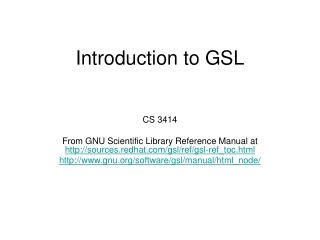DownloadDownload PresentationIntroduction to GSL

# Introduction to GSL

Télécharger la présentation## Introduction to GSL

- - - - - - - - - - - - - - - - - - - - - - - - - - - E N D - - - - - - - - - - - - - - - - - - - - - - - - - - -
##### Presentation Transcript

1. Introduction to GSL CS 3414 From GNU Scientific Library Reference Manual at http://sources.redhat.com/gsl/ref/gsl-ref_toc.html http://www.gnu.org/software/gsl/manual/html_node/

2. What is GSL? • The GNU Scientific Library • GNU is a recursive acronym for "GNU's Not Unix!”, a project started in 1983 by Richard Stallman • GNU is an operating system (HURD kernel) and an extensive collection of computer software • GSL is a numerical library for C and C++ programmers • GSL is free software distributed under the terms of the GNU General Public License • The combination of GNU software and the Linux kernel is commonly known as Linux

3. Functionality • GSL provides a well-defined C language Applications Programming Interface (API) for common numerical functions, such as: • Random Numbers • Least-Squares Fitting • Fast Fourier Transforms • Root-Finding • Minimization • Etc.http://www.gnu.org/software/gsl/manual/html_node/ • GSL is thread-safe (the library can be used in multi-threaded programs)

4. Compiling and Linking • The library header files are installed in their own “gsl” directory. You should write include statements with a “gsl/” directory prefix like: #include <gsl/gsl_math.h> • Compile: gcc -c example.c • Link: gcc example.o -lgsl -lgslcblas -lm

5. One dimensional Root-Finding • The header file `gsl_roots.h' contains prototypes for the root finding functions and related declarations. • The library provides low level components for a variety of iterative solvers and convergence tests. These can be combined by the user to achieve the desired solution, with full access to the intermediate steps of the iteration.

6. Root Finding Algorithms • Two classes of algorithms • root bracketing algorithms • begin with a bounded region known to contain a root • uses only function evaluations (not derivatives) • guaranteed to converge • root polishing algorithms • improve an initial guess to the root • require both the function and its derivative to be supplied by the user • converge only if started “close enough” to a root

7. Framework • The user provides a high-level driver for the algorithms. • The library provides the individual functions necessary for each of the steps. • There are three main phases of the iteration. The steps are, • initialize solver state, s, for algorithm T • update s using the iteration T • test s for convergence, and repeat iteration if necessary • The state for solver is held in a gsl_root_fsolverstruct (a declaration for a grouped list of variables in C) or in gsl_root_fdfsolverstruct.

8. Initializing the Solver • gsl_root_fsolver * gsl_root_fsolver_alloc(const gsl_root_fsolver_type * T ) • This function returns a pointer to a newly allocated instance of a solver of type T • void gsl_root_fsolver_free(gsl_root_fsolver * s) • This function frees all the memory associated with the solver s.

9. Providing the function to solve • Data Type:gsl_function • This data type defines a general function with parameters. • double (* function) (double x, void * params) • this function should return the value f(x,params) for argument x and parameters params • void * params • a pointer to the parameters of the function

10. Iteration • intgsl_root_fsolver_iterate(gsl_root_fsolver * s) • perform a single iteration of the solver to update its state s • double gsl_root_fsolver_root(constgsl_root_fsolver * s) • The solver maintains a current best estimate of the root at all times.

11. Search Stopping Parameters • A root finding procedure should stop when one of the following conditions is true: • A root has been found to within the user-specified precision. • A user-specified maximum number of iterations has been reached. • An error has occurred.

12. Precision Test • int gsl_root_test_interval(double x_lower, double x_upper, double epsabs, double epsrel) • |a - b| < epsabs + epsrel min(|a|,|b|)

13. Root Bracketing Algorithms • bisection algorithm - gsl_root_fsolver_bisection • false position algorithm - gsl_root_fsolver_falsepos • Brent-Dekker method - gsl_root_fsolver_brent

14. Root Finding Algorithms using Derivatives • Newton's Method - gsl_root_fdfsolver_newton • secant method (a simplified version of Newton's method which does not require the computation of the derivative on every step) - gsl_root_fdfsolver_secant • Steffenson Method - gsl_root_fdfsolver_steffenson

15. Resource • Read GNU Scientific Library Reference Manual at http://sources.redhat.com/gsl/ref/gsl-ref_toc.html